# Questions - Discrete Fourier Transform Notes | Study Digital Signal Processing - Electrical Engineering (EE)

## Electrical Engineering (EE): Questions - Discrete Fourier Transform Notes | Study Digital Signal Processing - Electrical Engineering (EE)

The document Questions - Discrete Fourier Transform Notes | Study Digital Signal Processing - Electrical Engineering (EE) is a part of the Electrical Engineering (EE) Course Digital Signal Processing.
All you need of Electrical Engineering (EE) at this link: Electrical Engineering (EE)

Questions

1 Q.  (i) {1,0,0,…….0} (impulse) ⇔ {1,1,1…..1} (constant)

(ii) {1,1,1,……1} (constant) ⇔) {N,0,0,&&.0} (impulse)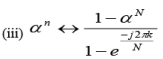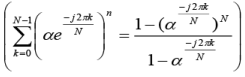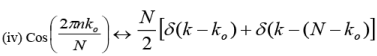(Impulse pair)

Or        Cos (2πnf ) = Cos (wn)

Sol.  x(n)  =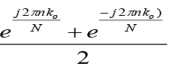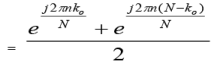We know that 1⇔ N δ (k )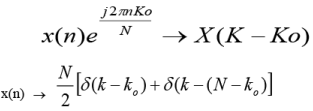I. Inverse DFT of a constant is a unit sample.
II. DFT of a constant is a unit sample.

2 Q. Find 10 point IDFT of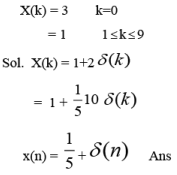3 Q. Suppose that we are given a program to find the DFT of a complex-valued sequence x(n). How can this program be used to find the inverse DFT of X(k)?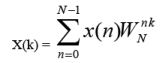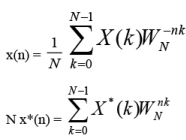1. Conjugate the DFT coefficients X(k) to produce the sequence X*(k).

2. Use the program to fing DFT of a sequence X*(k).

3. Conjugate the result obtained in step 2 and divide by N.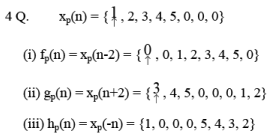5 Q.  x(n) = {1, 1, 0, 0, 0, 0, 0, 0}  n = 0 to 7 Find DFT.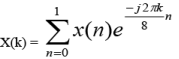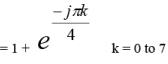X(0) = 1+1 = 2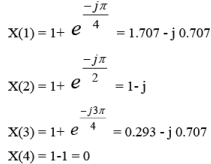By conjugate symmetry X(k) = X*(N-k) = X*(8-k)

∴X(5) = X*(3) = 0.293 + j 0.707

X(6) = X*(2) = 1+j

X(7) = X*(1) = 1.707 + j 0.707

X(k) = { ­2 , 1.707 - j 0.707, 0.293 - j 0.707, 1-j, 0, 1+j, 0.293 + j 0.707, 1.707 + j 0.707 }

6 Q. x(n) = {1, 2, 1, 0} N=4

X(k) = {4, -j2, 0, j2}

(i) y(n) = x(n-2) = {1, 0, 1, 2}

Y(k) = X(k) e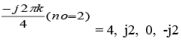(ii) X(k-1) = {j2, 4, -j2, 0}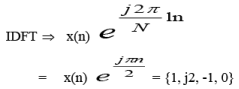(iii) g(n) = x(-n) = 1, 0, 1, 2

G(k) = X(-k) = X*(k) = {4, j2, 0, -j2}

(iv) p(n) = x*(n) = {1, 2, 1, 0}

P(k) = X*(-k) = {4, j2, 0, -j2}* = {4, -j2, 0, j2}

(v) h(n) = x(n) x(n)

= {1, 4, 1, 0}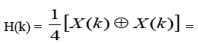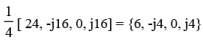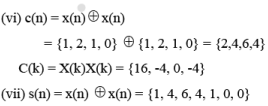S(k) = X(k) X(k) = {16, -2.35- j 10.28,  -2.18 + j 1.05, 0.02 + j 0.03, 0.02 - j 0.03, -2.18 -j 1.05,  -2.35 + j 10.28}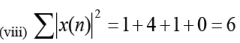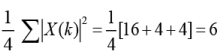The document Questions - Discrete Fourier Transform Notes | Study Digital Signal Processing - Electrical Engineering (EE) is a part of the Electrical Engineering (EE) Course Digital Signal Processing.
All you need of Electrical Engineering (EE) at this link: Electrical Engineering (EE)Use Code STAYHOME200 and get INR 200 additional OFF

## Digital Signal Processing

3 videos|50 docs|54 tests

Track your progress, build streaks, highlight & save important lessons and more!

,

,

,

,

,

,

,

,

,

,

,

,

,

,

,

,

,

,

,

,

,

;